Premium

# 4.NF.A.2 Worksheets, Workbooks, Lesson Plans, and Games

#### CCSS.maths.CONTENT.4.NF.A.2

:
"Compare two fractions with different numerators and different denominators, e.g., by creating common denominators or numerators, or by comparing to a benchmark fraction such as 1/2. recognise that comparisons are valid only when the two fractions refer to the same whole. Record the results of comparisons with symbols >, =, or

These worksheets can help students practise this Common Core State Standards skill.

## Worksheets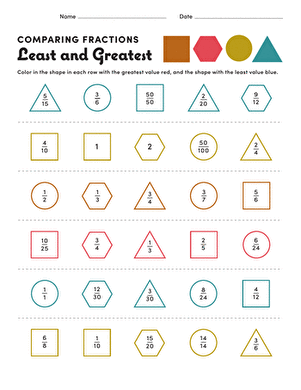Comparing Fractions: Least and Greatest
Worksheet
Comparing Fractions: Least and Greatest
Here's a maths challenge for your 4th grader: in this worksheet, your child will determine which fraction is greatest and which is least.
Year 5
Maths
WorksheetPractising Fractions: Odd Man Out
Worksheet
Practising Fractions: Odd Man Out
Here's a challenging maths worksheet for your 4th grader: in each set of fractions, your child must determine which is not equal to the others.
Year 5
Maths
Worksheet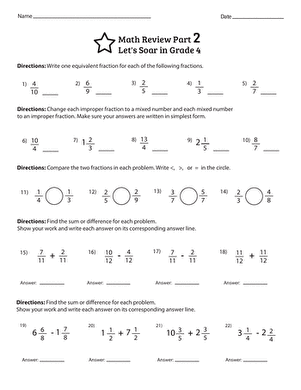Maths Review Part 2: Let's Soar in Grade 4
Worksheet
Maths Review Part 2: Let's Soar in Grade 4
Students show what they know about their fourth-grade fraction skills!
Year 5
Maths
Worksheet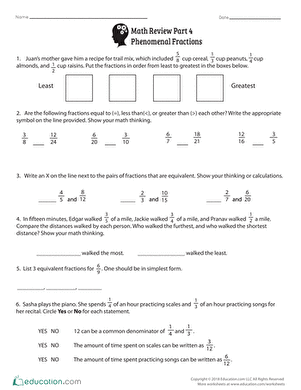Maths Review Part 4: Phenomenal Fractions
Worksheet
Maths Review Part 4: Phenomenal Fractions
Check your students’ mastery of year five concepts surrounding fractions!
Year 5
Maths
Worksheet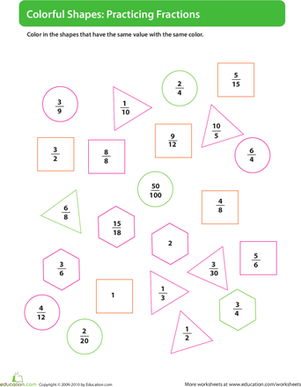Colour by Fraction
Worksheet
Colour by Fraction
Here's a challenging maths worksheet for your 4th grader: your child must colour the fractions according to their value, giving each value its own colour.
Year 5
Maths
Worksheet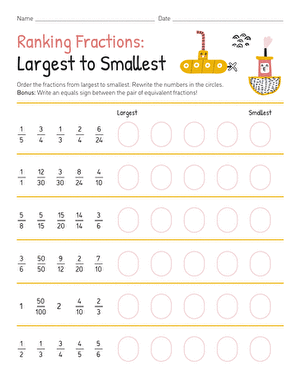Practising Fractions: Largest to Smallest
Worksheet
Practising Fractions: Largest to Smallest
Here's a challenging maths worksheet for your 4th grader: your child must put each set of fractions in order from greatest to least.
Year 5
Maths
Worksheet

## Lesson PlansFraction Wars
Lesson plan
Fraction Wars
Help kids master fractions with the fun, fast-paced, maths comparison game Fraction Wars. After a review, students are split into pairs to practise quickly comparing fractions with different numerators and denominators.
Year 5
Maths
Lesson planStrategies for Comparing Fractions
Lesson plan
Strategies for Comparing Fractions
Help your students see the variety of strategies they can use to compare fractions! Students will compare their strategies with that of their classmates. Use this lesson on its own or use it as support for the lesson Fraction Wars.
Year 5
Maths
Lesson plan

## Workbooks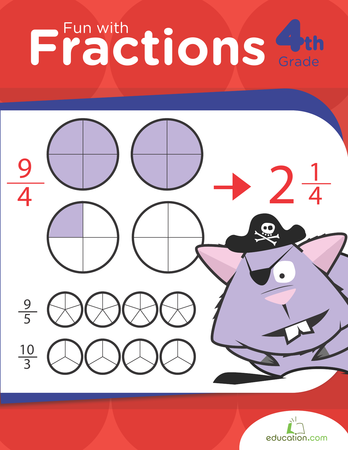Fun with Fractions
Workbook
Fun with Fractions
This fraction workbook covers fraction equivalents, simplification, adding and subtracting and improper fractions.
Year 5
Maths
Workbook

## Games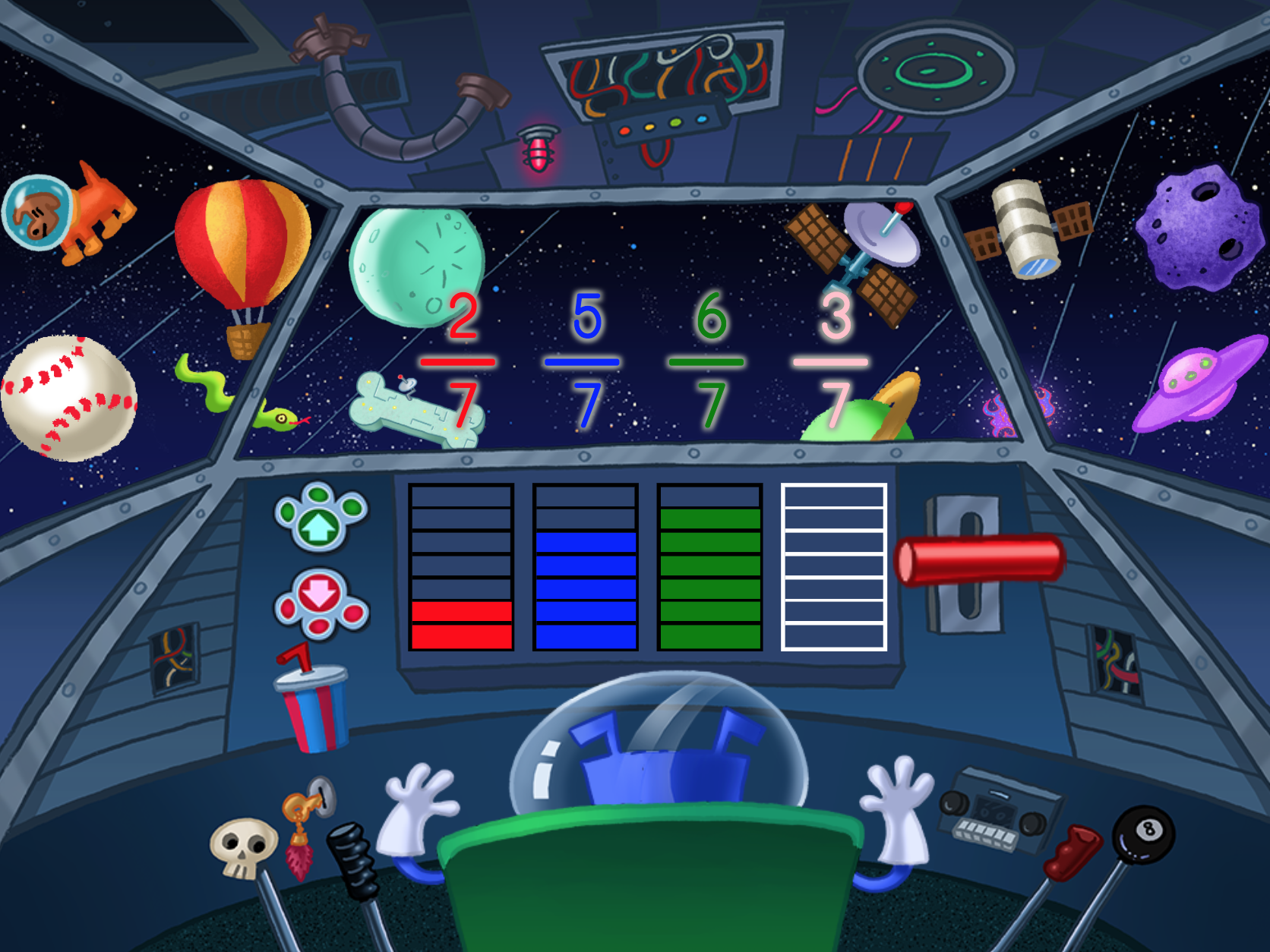Galactic Space Fractions: Comparing Like Denominators
Game
Galactic Space Fractions: Comparing Like Denominators
Kids use visual area models to compare fractions with common denominators in this intergalactic maths game.
Year 5
Maths
Game

## Exercises

No exercises found for this common core node.

### Add to collection

Create new collection

0

### New Collection>

0Items

What could we do to improve Education.com?

Please note: Use the Contact Us link at the bottom of our website for account-specific questions or issues.

What would make you love Education.com?